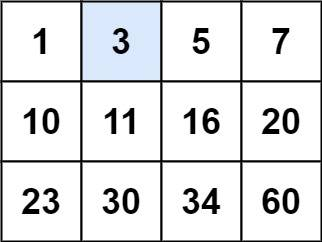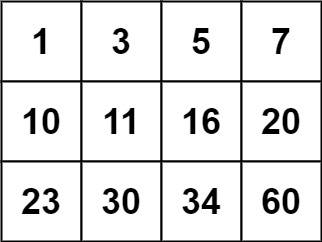# 2022-05-28

## ​190. Reverse Bits​

### Description

Reverse bits of a given 32 bits unsigned integer.
Note:
• Note that in some languages, such as Java, there is no unsigned integer type. In this case, both input and output will be given as a signed integer type. They should not affect your implementation, as the integer's internal binary representation is the same, whether it is signed or unsigned.
• In Java, the compiler represents the signed integers using 2's complement notation. Therefore, in Example 2 above, the input represents the signed integer `-3` and the output represents the signed integer `-1073741825`.
Example 1:
Input: n = 00000010100101000001111010011100
Output: 964176192 (00111001011110000010100101000000)
Explanation: The input binary string 00000010100101000001111010011100 represents the unsigned integer 43261596, so return 964176192 which its binary representation is 00111001011110000010100101000000.
Example 2:
Input: n = 11111111111111111111111111111101
Output: 3221225471 (10111111111111111111111111111111)
Explanation: The input binary string 11111111111111111111111111111101 represents the unsigned integer 4294967293, so return 3221225471 which its binary representation is 10111111111111111111111111111111.
Constraints:
• The input must be a binary string of length `32`
Follow up: If this function is called many times, how would you optimize it?

### Solution

#### Approach #0

func reverseBits(num uint32) uint32 {
var res uint32
for i := 0; i < 32 && num > 0; i++ {
res |= num & 1 << (31 - i)
num >>= 1
}
return res
}

#### Approach #1: Divide And Conquer

Without reading the official solution, this approach will never come into my mind...
const (
m1 = 0x55555555 // 01010101010101010101010101010101
m2 = 0x33333333 // 00110011001100110011001100110011
m4 = 0x0f0f0f0f // 00001111000011110000111100001111
m8 = 0x00ff00ff // 00000000111111110000000011111111
)
func reverseBits(n uint32) uint32 {
n = n>>1&m1 | n&m1<<1
n = n>>2&m2 | n&m2<<2
n = n>>4&m4 | n&m4<<4
n = n>>8&m8 | n&m8<<8
return n>>16 | n<<16
}

## ​136. Single Number​

### Description

Given a non-empty array of integers `nums`, every element appears twice except for one. Find that single one.
You must implement a solution with a linear runtime complexity and use only constant extra space.
Example 1:
Input: nums = [2,2,1]
Output: 1
Example 2:
Input: nums = [4,1,2,1,2]
Output: 4
Example 3:
Input: nums = 
Output: 1
Constraints:
• `1 <= nums.length <= 3 * 10^4`
• `-3 * 10^4 <= nums[i] <= 3 * 10^4`
• Each element in the array appears twice except for one element which appears only once.

### Solution

#### Approach #0

func singleNumber(nums []int) int {
res := 0
for _, num := range nums {
res ^= num
}
return res
}

## ​1021. Remove Outermost Parentheses​

### Description

A valid parentheses string is either empty `""`, `"(" + A + ")"`, or `A + B`, where `A` and `B` are valid parentheses strings, and `+` represents string concatenation.
• For example, `""`, `"()"`, `"(())()"`, and `"(()(()))"` are all valid parentheses strings.
A valid parentheses string `s` is primitive if it is nonempty, and there does not exist a way to split it into `s = A + B`, with `A` and `B` nonempty valid parentheses strings.
Given a valid parentheses string `s`, consider its primitive decomposition: `s = P1 + P2 + ... + Pk`, where `Pi` are primitive valid parentheses strings.
Return `s` after removing the outermost parentheses of every primitive string in the primitive decomposition of `s`.
Example 1:
Input: s = "(()())(())"
Output: "()()()"
Explanation:
The input string is "(()())(())", with primitive decomposition "(()())" + "(())".
After removing outer parentheses of each part, this is "()()" + "()" = "()()()".
Example 2:
Input: s = "(()())(())(()(()))"
Output: "()()()()(())"
Explanation:
The input string is "(()())(())(()(()))", with primitive decomposition "(()())" + "(())" + "(()(()))".
After removing outer parentheses of each part, this is "()()" + "()" + "()(())" = "()()()()(())".
Example 3:
Input: s = "()()"
Output: ""
Explanation:
The input string is "()()", with primitive decomposition "()" + "()".
After removing outer parentheses of each part, this is "" + "" = "".
Constraints:
• `1 <= s.length <= 105`
• `s[i]` is either `'('` or `')'`.
• `s` is a valid parentheses string.

### Solution

#### Approach #0

func removeOuterParentheses(s string) string {
var res, stack []rune
for _, ch := range s {
if ch == ')' {
stack = stack[:len(stack)-1]
}
if len(stack) > 0 {
res = append(res, ch)
}
if ch == '(' {
stack = append(stack, ch)
}
}
return string(res)
}

## ​34. Find First and Last Position of Element in Sorted Array​

### Description

Given an array of integers `nums` sorted in non-decreasing order, find the starting and ending position of a given `target` value.
If `target` is not found in the array, return `[-1, -1]`.
You must write an algorithm with `O(log n)` runtime complexity.
Example 1:
Input: nums = [5,7,7,8,8,10], target = 8
Output: [3,4]
Example 2:
Input: nums = [5,7,7,8,8,10], target = 6
Output: [-1,-1]
Example 3:
Input: nums = [], target = 0
Output: [-1,-1]
Constraints:
• `0 <= nums.length <= 10^5`
• `-10^9 <= nums[i] <= 10^9`
• `nums` is a non-decreasing array.
• `-10^9 <= target <= 10^9`

### Solution

#### Approach #0

func binarySearch(nums []int, target int, lower bool) (res int) {
res = len(nums)
for i, j := 0, len(nums)-1; i <= j; {
mid := (i + j) / 2
if nums[mid] > target || (lower && nums[mid] >= target) {
j = mid - 1
res = mid
} else {
i = mid + 1
}
}
return
}
func searchRange(nums []int, target int) []int {
low := binarySearch(nums, target, true)
high := binarySearch(nums, target, false) - 1
if low <= high && high < len(nums) && nums[low] == nums[high] {
return []int{low, high}
}
return []int{-1, -1}
}

#### Approach #1

func searchRange(nums []int, target int) []int {
low := sort.SearchInts(nums, target)
if low >= len(nums) || nums[low] != target {
return []int{-1, -1}
}
high := sort.SearchInts(nums, target+1) - 1
return []int{low, high}
}

## ​33. Search in Rotated Sorted Array​

### Description

There is an integer array `nums` sorted in ascending order (with distinct values).
Prior to being passed to your function, `nums` is possibly rotated at an unknown pivot index `k` (`1 <= k < nums.length`) such that the resulting array is `[nums[k], nums[k+1], ..., nums[n-1], nums, nums, ..., nums[k-1]]` (0-indexed). For example, `[0,1,2,4,5,6,7]` might be rotated at pivot index `3` and become `[4,5,6,7,0,1,2]`.
Given the array `nums` after the possible rotation and an integer `target`, return the index of `target` if it is in `nums`, or `-1` if it is not in `nums`.
You must write an algorithm with `O(log n)` runtime complexity.
Example 1:
Input: nums = [4,5,6,7,0,1,2], target = 0
Output: 4
Example 2:
Input: nums = [4,5,6,7,0,1,2], target = 3
Output: -1
Example 3:
Input: nums = , target = 0
Output: -1
Constraints:
• `1 <= nums.length <= 5000`
• `-10^4 <= nums[i] <= 10^4`
• All values of `nums` are unique.
• `nums` is an ascending array that is possibly rotated.
• `-10^4 <= target <= 10^4`

### Solution

#### Approach #0

func search(nums []int, target int) int {
n := len(nums)
if n == 0 {
return -1
}
if n == 1 {
if nums == target {
return 0
}
return -1
}
for i, j := 0, n-1; i <= j; {
mid := (i + j) / 2
if nums[mid] == target {
return mid
}
if nums <= nums[mid] {
if nums <= target && target < nums[mid] {
j = mid - 1
} else {
i = mid + 1
}
} else {
if nums[mid] < target && target <= nums[n-1] {
i = mid + 1
} else {
j = mid - 1
}
}
}
return -1
}

## ​74. Search a 2D Matrix​

### Description

Write an efficient algorithm that searches for a value `target` in an `m x n` integer matrix `matrix`. This matrix has the following properties:
• Integers in each row are sorted from left to right.
• The first integer of each row is greater than the last integer of the previous row.
Example 1:Input: matrix = [[1,3,5,7],[10,11,16,20],[23,30,34,60]], target = 3
Output: true
Example 2:Input: matrix = [[1,3,5,7],[10,11,16,20],[23,30,34,60]], target = 13
Output: false
Constraints:
• `m == matrix.length`
• `n == matrix[i].length`
• `1 <= m, n <= 100`
• `-10^4 <= matrix[i][j], target <= 10^4`

### Solution

#### Approach #0

func searchMatrix(matrix [][]int, target int) bool {
m, n := len(matrix), len(matrix)
i, j := -1, m-1
for i < j {
mid := (j-i+1)/2 + i
if matrix[mid] <= target {
i = mid
} else {
j = mid - 1
}
}
if i == -1 {
return false
}
for p, q := 0, n-1; p <= q; {
mid := (p-q)/2 + q
if matrix[i][mid] == target {
return true
}
if matrix[i][mid] > target {
q = mid - 1
} else {
p = mid + 1
}
}
return false
}

#### Approach #1

func searchMatrix(matrix [][]int, target int) bool {
m, n := len(matrix), len(matrix)
row := sort.Search(m, func(i int) bool { return matrix[i] > target }) - 1
if row < 0 {
return false
}
column := sort.SearchInts(matrix[row], target)
return column < n && matrix[row][column] == target
}

#### Approach #2

func searchMatrix(matrix [][]int, target int) bool {
m, n := len(matrix), len(matrix)
i := sort.Search(m*n, func(i int) bool { return matrix[i/n][i%n] >= target })
return i < m*n && matrix[i/n][i%n] == target
}